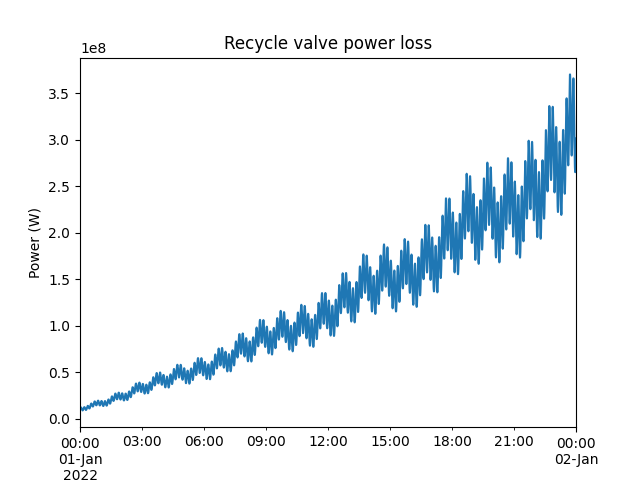# Pump recycle valve power loss

This example demonstrates how to calculate the recirculation line power loss if you have the following information:

• Pump suction pressure

• Pump discharge pressure

• Recycle valve outlet pressure

• Recycle valve flow coefficient ($$Cv$$) curve

• Density of the fluid

Note that if the flow rate through the recycle valve is known, the calculation can be simplified and the recycle valve outlet pressure and $$Cv$$ curve are not required.

We start by making some example dummy data. We ensure that the pump discharge pressure is higher than the suction pressure, and that the feed pressure is not constant to get more interesting results.

import pandas as pd

from indsl.equipment.valve_parameters import flow_through_valve
from indsl.signals.generator import line, sine_wave

start_date = pd.Timestamp("2022-1-1")
end_date = pd.Timestamp("2022-1-2")
mean_disch_P = 10  # bar
wave_period_disch_P = pd.Timedelta("10min")
wave_period_valve_out_P = pd.Timedelta("5hour")
suction_pressure = sine_wave(start_date, end_date)  # bar
discharge_pressure = sine_wave(start_date, end_date, wave_period=wave_period_disch_P, wave_mean=mean_disch_P)  # bar
valve_outlet_P = sine_wave(start_date, end_date, wave_period=wave_period_valve_out_P)  # bar
SG = 1
slope = pd.Timedelta("1s") / (end_date - start_date)
valve_opening = line(start_date, end_date, slope=slope, intercept=0)


To specify the valve $$Cv$$ curve, the type of curve has to be given and two points on the curve, the $$Cv$$ at min adn max flow rates.

type = "EQ"
min_opening = 0.1
max_opening = 0.9
min_Cv = 10
max_Cv = 90


The first step step is to calculate the flow through the recycle valve. We assume the pump discharge pressure is equal to the recycle valve inlet pressure.

Q_valve = flow_through_valve(
discharge_pressure, valve_outlet_P, valve_opening, SG, type, min_opening, max_opening, min_Cv, max_Cv, True
)  # m3/h


The second step is to calcualte the total head of the pump.

den = 1000 * SG  # kg/m3
den = line(start_date, end_date, intercept=den)  # kg/m3
discharge_pressure *= 100000  # Pa
suction_pressure *= 100000  # Pa


The last step is to calculate the recycle valve power loss.

power_loss = recycle_valve_power_loss(Q_valve, head, den, True)  # W
ax = power_loss.plot()
ax.set_title("Recycle valve power loss")
ax.set_xlabel("Time")
_ = ax.set_ylabel("Power (W)")As a simple sanity check, the increasing power loss with increasing valve opening makes sense, as this means more fluid flows through the recirculation line.

Total running time of the script: ( 0 minutes 0.543 seconds)

Gallery generated by Sphinx-Gallery